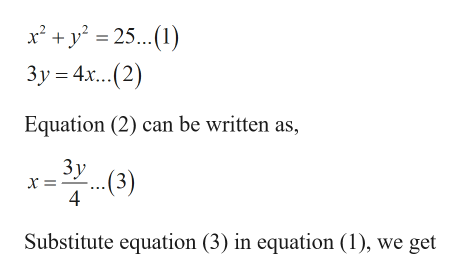# a. Graph the equations in the system. b. Solve the system by using the substitution method.x2 +y2 =253y=4x

Question
6 views

a. Graph the equations in the system. b. Solve the system by using the substitution method.

x+y=25

3y=4x

check_circle

Step 1

Given:

Step 2

Calculation:

a) To graph the equations:

Step 3

b) To solve the system of equations by su...help_outlineImage Transcriptionclosex' + y° = 25...(1) Зу%3 4х...(2) Equation (2) can be written as, Зу х- -(3) 4 Substitute equation (3) in equation (1), we get fullscreen

### Want to see the full answer?

See Solution

#### Want to see this answer and more?

Solutions are written by subject experts who are available 24/7. Questions are typically answered within 1 hour.*

See Solution
*Response times may vary by subject and question.
Tagged in

### Equations and In-equations